Define PDE Initial Condition VI

LabVIEW 2018 Help

Edition Date: March 2018
Part Number: 371361R-01
View Product InfoLabVIEW 2016 HelpLabVIEW 2017 HelpLabVIEW 2018 HelpLabVIEW 2019 HelpLabVIEW 2020 Help

Owning Palette: Partial Differential Equations VIs

Requires: Full Development System

Defines the initial condition of a partial differential equation. You must manually select the polymorphic instance to use.

Use the pull-down menu to select an instance of this VI.

 Select an instance 1D Heat (Initial Condition is Numeric)1D Heat (Initial Condition is VI)2D Heat (Initial Condition is Numeric)2D Heat (Initial Condition is VI)1D Wave (Initial Condition is Numeric)1D Wave (Initial Condition is VI)2D Wave (Initial Condition is Numeric)2D Wave (Initial Condition is VI)

1D Heat (Initial Condition is Numeric)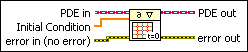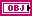PDE in is the class that stores the data of the equation.Initial Condition specifies the value of the initial condition. The length of Initial Condition must be # of x-points from the Define PDE Domain VI. Initial Condition stores the values of the unknown function evaluated on points X at initial t from the Define PDE Domain VI. By default, LabVIEW assumes that its values are zeros.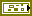error in describes error conditions that occur before this node runs. This input provides standard error in functionality.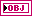PDE out returns PDE in with the initial condition.error out contains error information. This output provides standard error out functionality.

1D Heat (Initial Condition is VI)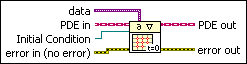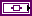data is a variant that passes arbitrary values to the VI.PDE in is the class that stores the data of the equation.Initial Condition is a strictly typed reference to the VI that implements the initial condition. Create this VI by starting from the VI template located in labview\vi.lib\gmath\pde.llb\Common\1D Evolutionary PDE Func Template.vit.error in describes error conditions that occur before this node runs. This input provides standard error in functionality.PDE out returns PDE in with the initial condition.error out contains error information. This output provides standard error out functionality.

2D Heat (Initial Condition is Numeric)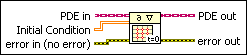PDE in is the class that stores the data of the equation.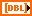Initial Condition specifies the value of the initial condition. The size of Initial Condition must be # of y-points-by-# of x-points from the Define PDE Domain VI. Each row or column of Initial Condition stores the values of the unknown function evaluated on a particular y- or x-point, respectively, at initial t from the Define PDE Domain VI.error in describes error conditions that occur before this node runs. This input provides standard error in functionality.PDE out returns PDE in with the initial condition.error out contains error information. This output provides standard error out functionality.

2D Heat (Initial Condition is VI)data is a variant that passes arbitrary values to the VI.PDE in is the class that stores the data of the equation.Initial Condition is a strictly typed reference to the VI that implements the initial condition. Create this VI by starting from the VI template located in labview\vi.lib\gmath\pde.llb\Common\2D Evolutionary PDE Func Template.vit.error in describes error conditions that occur before this node runs. This input provides standard error in functionality.PDE out returns PDE in with the initial condition.error out contains error information. This output provides standard error out functionality.

1D Wave (Initial Condition is Numeric)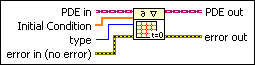PDE in is the class that stores the data of the equation.Initial Condition specifies the value of the initial condition.

The length of Initial Condition must be # of x-points from the Define PDE Domain VI. Initial Condition stores the values of the unknown function evaluated on points X at initial t from the Define PDE Domain VI. By default, LabVIEW assumes that its values are zeros.type specifies the type of the initial condition.

 0 Value (default)—The initial condition evaluates the unknown function at initial t from the Define PDE Domain VI. 1 Derivative—The initial condition evaluates the value of the partial derivative for the time variable at initial t from the Define PDE Domain VI.error in describes error conditions that occur before this node runs. This input provides standard error in functionality.PDE out returns PDE in with the initial condition.error out contains error information. This output provides standard error out functionality.

1D Wave (Initial Condition is VI)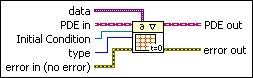data is a variant that passes arbitrary values to the VI.PDE in is the class that stores the data of the equation.Initial Condition is a strictly typed reference to the VI that implements the initial condition.

Create this VI by starting from the VI template located in labview\vi.lib\gmath\pde.llb\Common\1D Evolutionary PDE Func Template.vit.type specifies the type of the initial condition.

 0 Value (default)—The initial condition evaluates the unknown function at initial t from the Define PDE Domain VI. 1 Derivative—The initial condition evaluates the value of the partial derivative for the time variable at initial t from the Define PDE Domain VI.error in describes error conditions that occur before this node runs. This input provides standard error in functionality.PDE out returns PDE in with the initial condition.error out contains error information. This output provides standard error out functionality.

2D Wave (Initial Condition is Numeric)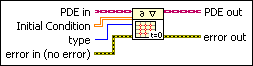PDE in is the class that stores the data of the equation.Initial Condition specifies the value of the initial condition. The size of Initial Condition must be # of y-points-by-# of x-points from the Define PDE Domain VI. Each row or column of Initial Condition stores the values of the unknown function evaluated on a particular y- or x-point, respectively, at initial t from the Define PDE Domain VI.type specifies the type of the initial condition.

 0 Value (default)—The initial condition evaluates the unknown function at initial t from the Define PDE Domain VI. 1 Derivative—The initial condition evaluates the value of the partial derivative for the time variable at initial t from the Define PDE Domain VI.error in describes error conditions that occur before this node runs. This input provides standard error in functionality.PDE out returns PDE in with the initial condition.error out contains error information. This output provides standard error out functionality.

2D Wave (Initial Condition is VI)data is a variant that passes arbitrary values to the VI.PDE in is the class that stores the data of the equation.Initial Condition is a strictly typed reference to the VI that implements the initial condition.

Create this VI by starting from the VI template located in labview\vi.lib\gmath\pde.llb\Common\2D Evolutionary PDE Func Template.vit.type specifies the type of the initial condition.

 0 Value (default)—The initial condition evaluates the unknown function at initial t from the Define PDE Domain VI. 1 Derivative—The initial condition evaluates the value of the partial derivative for the time variable at initial t from the Define PDE Domain VI.error in describes error conditions that occur before this node runs. This input provides standard error in functionality.PDE out returns PDE in with the initial condition.error out contains error information. This output provides standard error out functionality.

Define PDE Initial Condition Details

The heat equation requires only the initial value to solve the equation. However, for the wave equation, specify the value and the derivative value with respect to the time variable at initial time. The following block diagram is an example of how to define the initial condition for a one-dimensional wave equation. The VI defines the initial value, and the numeric array defines the initial derivative.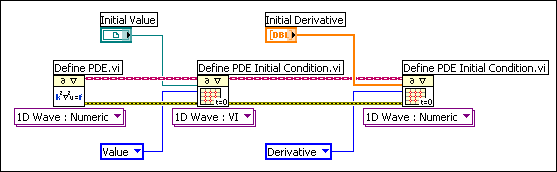Examples

Refer to the following VIs for examples of using the Define PDE Initial Condition VI:

• PDE String Vibration VI: labview\examples\Mathematics\Differential Equations - PDE
• PDE Thermal Distribution VI: labview\examples\Mathematics\Differential Equations - PDE